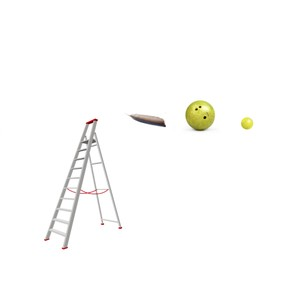Gravity and motion

# Gravity and motion

I can describe and predict the relationship between gravity and the motion of...

No account needed.8,000 schools use Gynzy92,000 teachers use Gynzy1,600,000 students use Gynzy

## General

In this lesson, students will look at how the forces of gravity affect the motion of objects. They will learn all about how objects fall and accelerate.

NGSS:
MS-PS2-2
MS-PS2-4

## Learning objective

Students will be able to describe and predict the relationship between gravity and the motion of an object.

## Introduction

Students will review the concept of gravity. They will also discuss which object (baseball, tennis ball, feather) when dropped from the same height simultaneously will hit the ground first.

## Instruction

Talk to students about velocity and acceleration. Explain how the force of gravity causes objects to fall toward the ground at the same rate. Use the diagram in the lesson for a visual representation. Students will calculate the velocity of a golf ball in a practice problem.

Next, explain that air resistance is a force that opposes the motion of objects as they move through the air. The lesson will return to the feather from the introduction and why it hit the ground last.

Explain that terminal velocity is the maximum velocity that an object reaches when it is falling. Students will look at this concept with the example of parachutes.

## Quiz

Students will respond to ten multiple-choice and short-answer questions.

## Closing

Students will recall what they learned in the lesson and complete an exit slip.

### The online teaching platform for interactive whiteboards and displays in schools

• Save time building lessons

• Manage the classroom more efficiently

• Increase student engagement